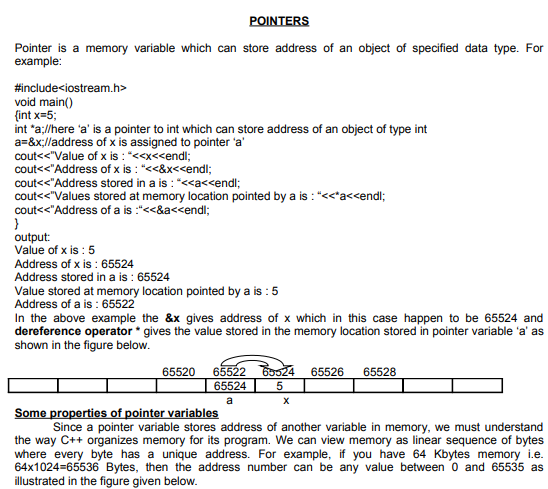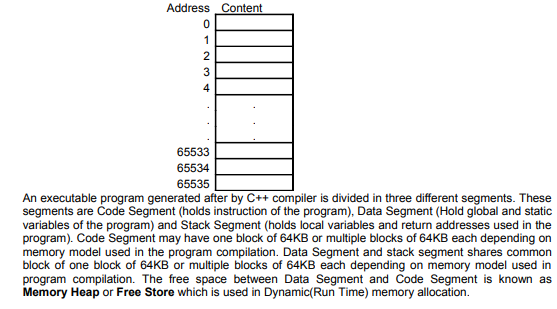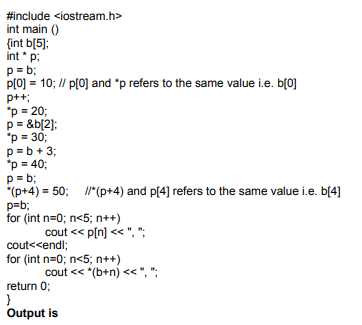# CBSE Class 12 Computer Science Pointers Notes

Download CBSE Class 12 Computer Science Pointers Notes in PDF format. All Revision notes for Class 12 Computer Science have been designed as per the latest syllabus and updated chapters given in your textbook for Computer Science in Class 12. Our teachers have designed these concept notes for the benefit of Class 12 students. You should use these chapter wise notes for revision on daily basis. These study notes can also be used for learning each chapter and its important and difficult topics or revision just before your exams to help you get better scores in upcoming examinations, You can also use Printable notes for Class 12 Computer Science for faster revision of difficult topics and get higher rank. After reading these notes also refer to MCQ questions for Class 12 Computer Science given on studiestoday

## Revision Notes for Class 12 Computer Science Pointers

Class 12 Computer Science students should refer to the following concepts and notes for Pointers in Class 12. These exam notes for Class 12 Computer Science will be very useful for upcoming class tests and examinations and help you to score good marks

### Pointers Notes Class 12 Computer Science10, 20, 30, 40, 50,

10, 20, 30, 40, 50,

Note: A pointer variable can be used as an array as in above example both *p and p refers to the same variable similarly b and *b refers to the same variable. Again p and *(p+1) are same.

Address calculation method is same for both pointer and array. For example int a={2,4,6,7,9};

int *q=a;

The address of a[index] is calculated as

Base address of a (i.e. address of a) + index * sizeof (data type of a)

for example if the base address of a is 1000 then address of a is calculated as

&a =1000 + 3 * sizeof (int) = 1000 + 3 * 2=1000 + 6 = 1006

Note that a and *&a both will give the same value i.e. 7

Similarly address of (q+3) is calculated as

Address stored in q + 3 * sizeof (data type of pointer belongs to in this case int) (q+3) = 1000 + 3 * sizeof (int) = 1000 + 3 * 2=1006

Arithmetic operation on pointers

We can increment or decrement a pointer variable for example float a={3.0,5.6,6.0,2.5,5.3};

float *p=a;

++p;

--p;

if the base address of a is 1000 then statement ++p will increment the address stored in pointer variable by 4 because p is a pointer to float and size of a float object is 4 byte, since ++p is equivalent to p=p+1 and address of p+1 is calculated as

Address stored in p + 1 * sizeof (float)= 1000 + 1 * 4 = 1004.

We can add or subtract any integer number to a pointer variable because adding an integer value to an address makes another address e.g.

void main()

{int p={8,6,4,2,1}; Int *q;

q=p;//address of p is assigned to q assuming p is allocated at memory location 1000 q=q+4;//now q contains address of p i.e. 1000+4*sizeof(int)=1000+4*2= 1008

cout<<*q<<endl;

q=q-2;//now q contains address of p i.e. 1008-2*sizeof (int)=1008-2*2=1004 cout<<*q<<endl;

}

Output is

1

4

Addition of two pointer variables is meaningless.

Subtraction of two pointers is meaningful only if both pointers contain the address of different elements of the same array

For example

void main()

{int p={1,3,5,6,8,9};

int *a=p+1,*b=p+4;

p=p+q; //error because sum of two addresses yields an illegal address int x=b-a; //valid

cout<<x;

}

Output is

3

In the above example if base address of a is 1000 then address of a would be 1002 and address of b would be 1008 then value of b-a is calculated as

(Address stored in b-address stored in a)/sizeof(data type in this case int) (1008-1002)/2=3

Multiplication and division operation on a pointer variable is not allowed

We cannot assign any integer value other than 0 to a pointer variable. For example

int *p;

p=5; //not allowed

p=0; //allowed because 0 is a value which can be assigned to a variable of any data type

Null Pointer

A null pointer is a regular pointer of any pointer type which has a special value that indicates that it is not pointing to any valid reference or memory address. This value is the result of type-casting the

integer value zero to any pointer type.

int *q=0; // q has a NULL pointer value float * p;

p=NULL; // p has a NULL (NULL is defined as a constant whose value is 0) pointer value

Note: 0 memory address is a memory location which is not allocated to any variable of the program.

voidpointers

void pointer is a special pointer which can store address of an object of any data type. Since void pointer do not contain the data type information of the object it is pointing to we can not apply dereference operator * to a void pointer without using explicit type casting.

void main()

{

int x=5;

float y=3.5;

int *p;

float *q;

void *r;

p=&y; //error cannot convert float * to int * q=&x; //error cannot convert int * to float * p=&x; //valid

cout<<*p <<endl; //valid q=&y; //valid

cout<<*q<<endl ; //valid

r=&x; //valid

cout<<*r<<endl; //error pointer to cannot be dereference without explicit type cast cout<<*(int *)r<<endl; // valid and display the values pointed by r as int

r=&y; //valid

cout<<*(float *)r<<endl; //valid and display the values pointed by r as float

}

Note: Pointer to one data type cannot be converted to pointer to another data type except pointer to void

Array of pointers and pointer to array

An array of pointer is simply an array whose all elements are pointers to the same data type.
For example

Void main()

{

float x=3.5,y=7.2,z=9.7;

float *b; // here b is an array of 5 pointers to float b=&x;

b=&y;

b=&z;

cout<<*b<<”\t”<<*b<<”\t”<<*b<<endl;

## More Study Material

### CBSE Class 12 Computer Science Pointers Notes

We hope you liked the above notes for topic Pointers which has been designed as per the latest syllabus for Class 12 Computer Science released by CBSE. Students of Class 12 should download and practice the above notes for Class 12 Computer Science regularly. All revision notes have been designed for Computer Science by referring to the most important topics which the students should learn to get better marks in examinations. Studiestoday is the best website for Class 12 students to download all latest study material.

### Notes for Computer Science CBSE Class 12 Pointers

Our team of expert teachers have referred to the NCERT book for Class 12 Computer Science to design the Computer Science Class 12 notes. If you read the concepts and revision notes for one chapter daily, students will get higher marks in Class 12 exams this year. Daily revision of Computer Science course notes and related study material will help you to have a better understanding of all concepts and also clear all your doubts. You can download all Revision notes for Class 12 Computer Science also from www.studiestoday.com absolutely free of cost in Pdf format. After reading the notes which have been developed as per the latest books also refer to the NCERT solutions for Class 12 Computer Science provided by our teachers

#### Pointers Notes for Computer Science CBSE Class 12

All revision class notes given above for Class 12 Computer Science have been developed as per the latest curriculum and books issued for the current academic year. The students of Class 12 can rest assured that the best teachers have designed the notes of Computer Science so that you are able to revise the entire syllabus if you download and read them carefully. We have also provided a lot of MCQ questions for Class 12 Computer Science in the notes so that you can learn the concepts and also solve questions relating to the topics. All study material for Class 12 Computer Science students have been given on studiestoday.

#### Pointers CBSE Class 12 Computer Science Notes

Regular notes reading helps to build a more comprehensive understanding of Pointers concepts. notes play a crucial role in understanding Pointers in CBSE Class 12. Students can download all the notes, worksheets, assignments, and practice papers of the same chapter in Class 12 Computer Science in Pdf format. You can print them or read them online on your computer or mobile.

#### Notes for CBSE Computer Science Class 12 Pointers

CBSE Class 12 Computer Science latest books have been used for writing the above notes. If you have exams then you should revise all concepts relating to Pointers by taking out a print and keeping them with you. We have also provided a lot of Worksheets for Class 12 Computer Science which you can use to further make yourself stronger in Computer Science

Where can I download latest CBSE Class 12 Computer Science Pointers notes

Can I download the Notes for Pointers Class 12 Computer Science in Pdf format

Yes, you can click on the link above and download notes PDFs for Class 12 Computer Science Pointers which you can use for daily revision

Are the revision notes available for Pointers Class 12 Computer Science for the latest CBSE academic session

Yes, the notes issued for Class 12 Computer Science Pointers have been made available here for latest CBSE session

How can I download the Pointers Class 12 Computer Science Notes pdf

You can easily access the link above and download the Class 12 Notes for Computer Science Pointers for each topic in Pdf

Is there any charge for the Class 12 Computer Science Pointers notes

There is no charge for the notes for CBSE Class 12 Computer Science Pointers, you can download everything free of charge

Which is the best online platform to find notes for Pointers Class 12 Computer Science

www.studiestoday.com is the best website from which you can download latest notes for Pointers Computer Science Class 12

Where can I find topic-wise notes for Class 12 Computer Science Pointers

Come to StudiesToday.com to get best quality topic wise notes for Class 12 Computer Science Pointers

Can I get latest Pointers Class 12 Computer Science revision notes as per CBSE syllabus

We have provided all notes for each topic of Class 12 Computer Science Pointers as per latest CBSE syllabus